# Discharge characteristics of electric double-layer capacitors manufactured by Vishay Corporation

To drive the Raspberry Pi with an electric double layer capacitor, we examined the discharge characteristics.

## At first

Because the voltage and the capacity are described in the specification of the electric double layer capacitor, it is easy to calculate the working time from there, but the actual behavior might be different from the one to expect it and examined it.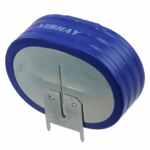We used the Vshay 196 HVC ENYCAP series electric double layer capacitor MAL219690102E3.

Since the spec is 5.6 V 90F, the calculation is likely to be stored in 90 * 5.6 ^ 2/2 = 1411J energy.

On the other hand, the value shown in the data sheet remains in the value of about 1/3 in 460J.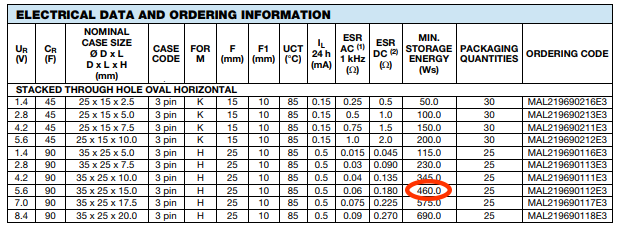We will examine how the difference of this value appears as a behavior in the future.

## Constant Current discharge

The transition of the voltage when the constant current discharge of 210 Ma is shown below.The measured values are green and the theoretical values are shown in orange.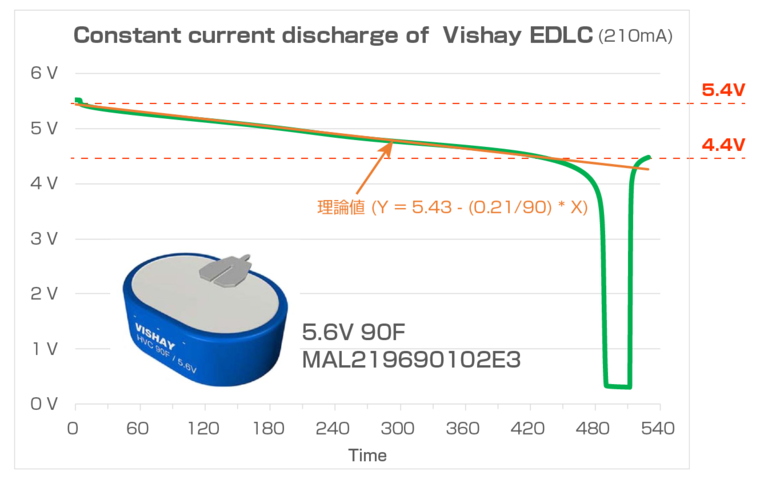From this graph, you can read the following three points.

• Match the theoretical value until the voltage is 4.4 V
• The discharged energy is almost identical to the spec.
• The internal resistance of the discharge endgame is quite large.

### Match the theoretical value until the voltage is 4.4 V

The formula for the theoretical value is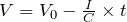, but it is almost identical until the voltage is 4.4 V.The discharge voltage on the specification is 3.2 V (0.8 V * 4cell), but it seems that there is a big change in behavior in front of 1V or more.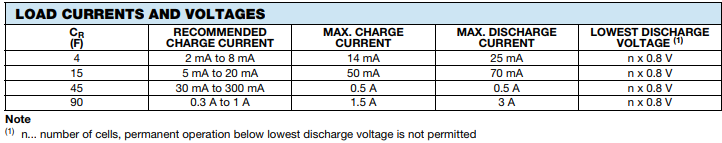### The discharged energy is almost identical to the spec.

Since the time it took to discharge from 5.4 V to 4.4 V is 450 seconds, the discharged energy is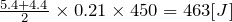. This is almost identical to the 460J described in the data sheet.

It's almost like a datasheet.

### The internal resistance of the discharge endgame is quite large.

When the discharge is stopped after the voltage becomes almost 0V (exactly 0.3 V), the voltage is rapidly recovering to 4.5 V.This shows that the internal resistance at this point has increase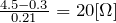.

The model of ELDC has been proposed as shown below, but it is said that the bottom Rn is roughly 20Ω.

## Constant Power discharge

Next, the transition of the voltage when it is discharged with a constant power of 1.05 W is shown below assuming the actual use case.The measurement was done by connecting the ELDC to a constant current load of 210 Ma via a SW power supply of 5V output.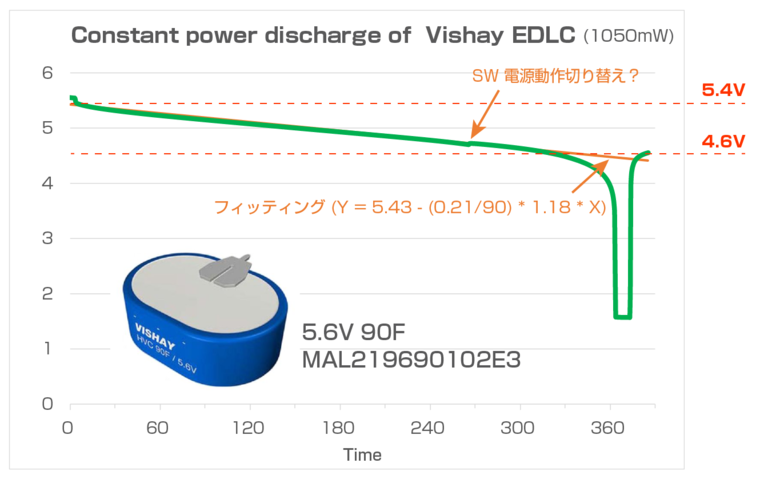From this graph, you can read the following two points.

• Clean properties until the voltage is 4.6 V
• The discharged energy is almost identical to the efficiency of the SW power supply.

### Clean properties until the voltage is 4.6 V

By discharging with constant power, the current value increases as the discharge progresses, but still the clean characteristics are maintained until 4.6 V as well as the constant current discharge.

It is thought that the deterioration of the characteristic worse than the time of 4.4 V at the time of constant current is due to the increase in the current value, which is susceptible to the influence of internal resistance.

### The discharged energy is almost identical to the efficiency of the SW power supply.

Since the time it took to discharge from 5.4 V to 4.6 V is 320 seconds, the discharged energy is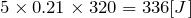. This corresponds to about 73% in the case of constant current discharge.

Because the efficiency of the SW power supply IC (LT3580) used at this time is about 73% in the buck and 78% by boosting, most of this change is explained by the efficiency of SW power supply.

## Bonus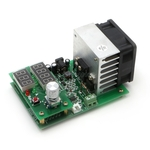When evaluating an electric double layer capacitor, it is recommended to use an electronic load.

The threshold is a little high, but AliExpress and left ones are available in less than \$20.There are many other things to search for in "Electronic Load".

It's cheap, but it works fine.The current value when a constant current discharge is made in the setting of 200mA is as follows.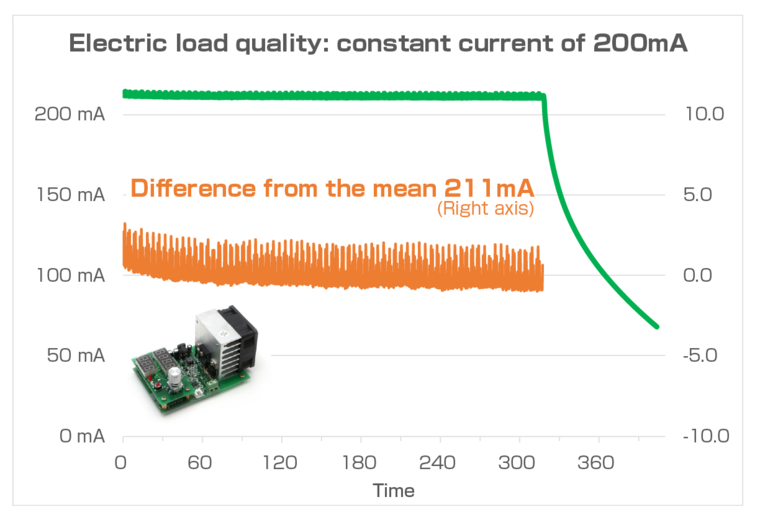There is an offset error, but the other is clean behavior.(This is because the electric double layer capacitor has been emptied in the end.)

## Summary

The power that can be stored in an electric double layer capacitor is very different from the one calculated in the spec C and V.
Because the value is described in the separate item of the datasheet, it seems to be good to refer to it.

シェアする
kimataをフォローするRabbit Note